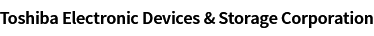### Cross Reference Search

About information presented in this cross reference

The information presented in this cross reference is based on TOSHIBA's selection criteria and should be treated as a suggestion only. Please carefully review the latest versions of all relevant information on the TOSHIBA products, including without limitation data sheets and validate all operating parameters of the TOSHIBA products to ensure that the suggested TOSHIBA products are truly compatible with your design and application.
Please note that this cross reference is based on TOSHIBA's estimate of compatibility with other manufacturers' products, based on other manufacturers' published data, at the time the data was collected.
TOSHIBA is not responsible for any incorrect or incomplete information. Information is subject to change at any time without notice.

### Stock Check & Purchase

Select Product Categories

Select Application

Find everything you need for your next product design. Simply select an application and click through to the block diagram to discover our semiconductor solutions.

New Products / News

Design & Development

Knowledge

# Calculating the Operating Supply Current and Power Dissipation

Calculating the power dissipation of a CMOS logic IC

Power dissipation should be calculated from both of the following:

• Static supply current
• Dynamic supply current

Power can be obtained by multiplying current by the voltage applied to an IC.

Static power dissipation: PS
While CMOS logic is in a static state (i.e., while its input current remains almost unchanged), little current flows in it except tiny leakage current that flows across the internal reverse-biased pn junction (known as static supply current, ICC). Static power dissipation is ICC multiplied by the supply voltage.
PS = VCC x ICC
VCC: Voltage applied to a logic IC
ICC: Static supply current shown in the datasheet

Dynamic power dissipation: PL+PPD
Dynamic supply current is the current that flows in CMOS logic ICs while its input transitions between High and Low. This current flows during the charging and discharging of capacitance. It is necessary to consider both parasitic capacitance (internal equivalent capacitance) and load capacitance.
Dynamic power dissipation is dynamic supply current multiplied by the voltage applied to the p-channel or n-channel MOSFET.
For the sake of simplicity, the following calculation assumes that this voltage is equal to VCC at which dynamic supply current becomes the maximum.

Dynamic power dissipation due to load capacitance (CL): PL
PL means power dissipation when an external load is charged and discharged as shown by the right-hand figure.
The amount of charge (QL) stored on the load capacitance is calculated as follows:
QL = CL * VCC
Let the output signal frequency be fOUT (= 1/TOUT). Then, the average current (IL) is expressed as follows:
IL = QL / T = CL * VCC * fOUT
Hence, dynamic power dissipation (PL) is:
PL = VCC * IL ＝ CL * VCC^2 * fOUT
If an IC has multiple outputs, its dynamic power dissipation can be calculated as follows:
PL = VCC^2 * Σ (CLn* fOUTn)

Dynamic power dissipation due to internal equivalent capacitance (CPD): PPD
CMOS logic ICs have various parasitic capacitances as shown by the right-hand figure. These capacitances are equivalently expressed as CPD. (Actually, CPD is calculated from power dissipation at relatively high frequency (1 MHz) under a zero-load condition.)
PPD is the power dissipated by the equivalent capacitance of an IC and can be considered in the same manner as PL.
Note, however, that PPD is calculated at input frequency (fIN):
PPD ＝ VCC * IL ＝ CPD * VCC^2 * fIN

Total power dissipation (PTTL) can be obtained as the sum of static power dissipation (PS) and dynamic power dissipation (PL + PPD):
PTTL＝ PS + PL + PPD

## Related information

• Application Notes
• FAQ
A new window will open
Site Map

Semiconductor Products

Storage Products

Other Products

Company